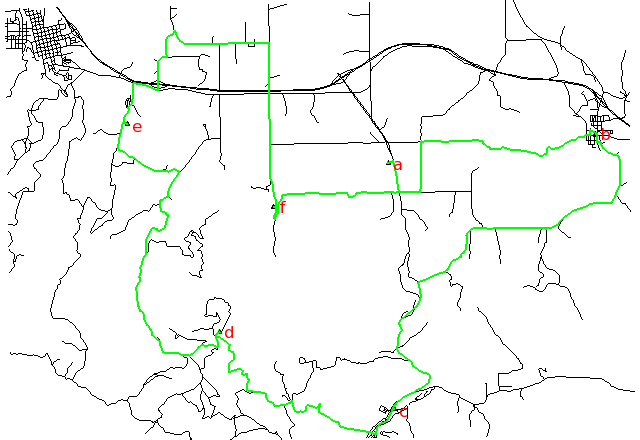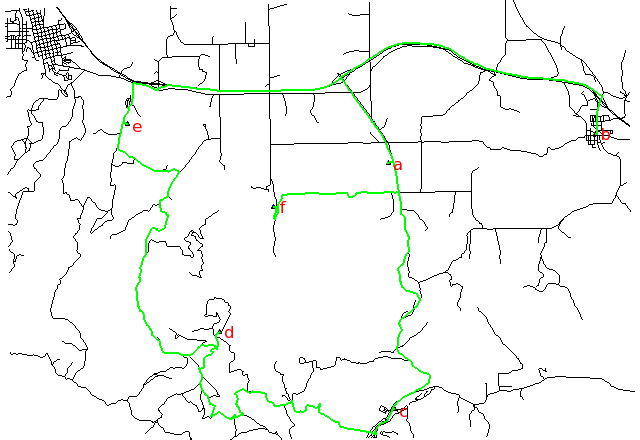## NAME

v.net.salesman - Creates a cycle connecting given nodes (Traveling salesman problem).
Note that TSP is NP-hard, heuristic algorithm is used by this module and created cycle may be sub optimal

## KEYWORDS

vector, network, salesman

## SYNOPSIS

v.net.salesman
v.net.salesman --help
v.net.salesman [-tg] input=name output=name center_cats=range arc_layer=string arc_type=string[,string,...] node_layer=string [arc_column=string] [arc_backward_column=string] [turn_layer=string] [turn_cat_layer=string] [sequence=name] [--overwrite] [--help] [--verbose] [--quiet] [--ui]

### Flags:

-t
Use turntable
-g
Use geodesic calculation for longitude-latitude locations
--overwrite
Allow output files to overwrite existing files
--help
Print usage summary
--verbose
Verbose module output
--quiet
Quiet module output
--ui
Force launching GUI dialog

### Parameters:

input=name [required]
Name of input vector map
Or data source for direct OGR access
output=name [required]
Name for output vector map
center_cats=range [required]
Category values
Categories of points ('cities') on nodes (layer is specified by nlayer)
arc_layer=string [required]
Arc layer
Vector features can have category values in different layers. This number determines which layer to use. When used with direct OGR access this is the layer name.
Default: 1
arc_type=string[,string,...] [required]
Arc type
Input feature type
Options: line, boundary
Default: line,boundary
node_layer=string [required]
Node layer (used for cities)
Vector features can have category values in different layers. This number determines which layer to use. When used with direct OGR access this is the layer name.
Default: 2
arc_column=string
Arc forward/both direction(s) cost column (number)
arc_backward_column=string
EXPERIMENTAL: Arc backward direction cost column (number)
turn_layer=string
Layer with turntable
Relevant only with -t flag
Default: 3
turn_cat_layer=string
Layer with unique categories used in turntable
Relevant only with -t flag
Default: 4
sequence=name
Name for output file holding node sequence ("-" for stdout)

## DESCRIPTION

v.net.salesman calculates the optimal route to visit nodes on a vector network.

Costs may be either line lengths, or attributes saved in a database table. These attribute values are taken as costs of whole segments, not as costs to traverse a length unit (e.g. meter) of the segment. For example, if the speed limit is 100 km / h, the cost to traverse a 10 km long road segment must be calculated as
length / speed = 10 km / (100 km/h) = 0.1 h.
Supported are cost assignments for arcs, and also different costs for both directions of a vector line. For areas, costs will be calculated along boundary lines.

The input vector needs to be prepared with v.net operation=connect in order to connect points representing center nodes to the network.

Points specified by category must be exactly on network nodes, and the input vector map needs to be prepared with v.net operation=connect.

Application of flag -t enables a turntable support. This flag requires additional parameters turn_layer and turn_cat_layer that are otherwise ignored. The turntable allows to model e.g. traffic code, where some turns may be prohibited. This means that the input layer is expanded by turntable with costs of every possible turn on any possible node (intersection) in both directions. Turntable can be created by the v.net module. For more information about turns in the vector network analyses see wiki page.

## NOTES

Arcs can be closed using cost = -1. Turns support: The costs of turns on visiting nodes are not taken in account.

## EXAMPLE

Traveling salesman for 6 digitized nodes (Spearfish):Fastest path, along highways:Searching for the shortest path using distance and the fastest path using traveling time according to the speed limits of different road types:

```# Spearfish

# we have 6 locations to visit on our trip
echo "1|601653.5|4922869.2|a
2|608284|4923776.6|b
3|601845|4914981.9|c
4|596270|4917456.3|d
5|593330.8|4924096.6|e
6|598005.5|4921439.2|f" | v.in.ascii in=- cat=1 x=2 y=3 out=centers col="cat integer, \
east double precision, north double precision, label varchar(43)"

# verify data preparation
v.db.select centers
v.category centers op=report
# type       count        min        max
# point          6          1          6

# create lines map connecting points to network (on layer 2)
# Layer / table: 1 / myroads_net
# type       count        min        max
# line         837          1          5
#
# Layer: 2
# type       count        min        max
# point          6          1          5

# find the shortest path

# set up costs as traveling time

# create unique categories for each road in layer 3

# add new table for layer 3
v.db.addtable myroads_net_time layer=3 col="cat integer,label varchar(43),length double precision,speed double precision,cost double precision,bcost double precision"

# copy road type to layer 3
v.to.db myroads_net_time layer=3 qlayer=1 opt=query qcolumn=label columns=label

v.to.db myroads_net_time layer=3 type=line option=length col=length unit=miles

# set speed limits in miles / hour
v.db.update myroads_net_time layer=3 col=speed val="75.0" where="label='interstate'"
v.db.update myroads_net_time layer=3 col=speed val="75.0" where="label='primary highway, hard surface'"
v.db.update myroads_net_time layer=3 col=speed val="50.0" where="label='secondary highway, hard surface'"

# define traveling costs as traveling time in minutes:

# set forward costs
v.db.update myroads_net_time layer=3 col=cost val="length / speed * 60"
# set backward costs
v.db.update myroads_net_time layer=3 col=bcost val="length / speed * 60"

# find the fastest path
v.net.salesman myroads_net_time arc_layer=3 node_layer=2 arc_column=cost arc_backward_column=bcost center_cats=1-6 out=mysalesman_time
```
To display the result, run for example:
```# Display the results

# shortest path
d.mon x0
d.vect centers -c icon=basic/triangle
d.vect mysalesman_distance col=green width=2
d.font Vera
d.vect centers col=red disp=attr attrcol=label lsize=12

# fastest path
d.mon x1
d.vect centers -c icon=basic/triangle
d.vect mysalesman_time col=green width=2
d.font Vera
d.vect centers col=red disp=attr attrcol=label lsize=12
```

d.path, v.net, v.net.alloc, v.net.iso, v.net.path, v.net.steiner